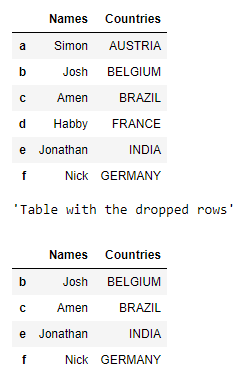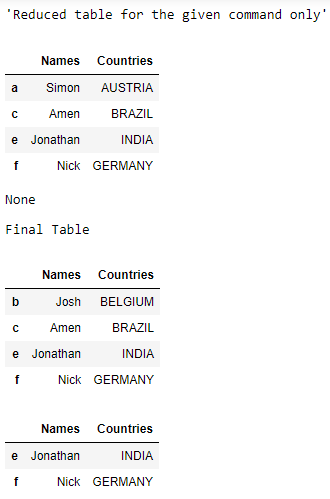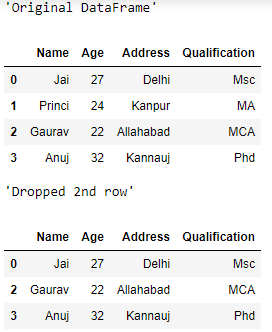Skip to content
Related Articles
Drop a list of rows from a Pandas DataFrame
• Last Updated : 17 Aug, 2020

Let us see how to drop a list of rows in a Pandas DataFrame. We can do this using the `drop()` function. We will also pass `inplace = True` as it makes sure that the changes we make in the instance are stored in that instance without doing any assignment
Over here is the code implementation of how to drop list of rows from the table :

Example 1 :

 `# imnport the module``import` `pandas as pd``  ` `# creating a DataFrame``dictionary ``=` `{``'Names'``:[``'Simon'``, ``'Josh'``, ``'Amen'``, ``'Habby'``, ``                       ``'Jonathan'``, ``'Nick'``], ``              ``'Countries'``:[``'AUSTRIA'``, ``'BELGIUM'``, ``'BRAZIL'``, ``                           ``'FRANCE'``, ``'INDIA'``, ``'GERMANY'``]}``table ``=` `pd.DataFrame(dictionary, columns ``=` `[``'Names'``, ``'Countries'``], ``                     ``index ``=` `[``'a'``, ``'b'``, ``'c'``, ``'d'``, ``'e'``, ``'f'``])``  ` `display(table)``  ` `# gives the table with the dropped rows``display(``"Table with the dropped rows"``)``display(table.drop([``'a'``, ``'d'``]))``  ` `# gives the table with the dropped rows ``# shows the reduced table for the given command only``display(``"Reduced table for the given command only"``)``display(table.drop(table.index[[``1``, ``3``]]))``  ` `# it gives none but it makes changes in the table ``display(table.drop([``'a'``, ``'d'``], inplace ``=` `True``))``  ` `# final table``print``(``"Final Table"``)``display(table)``  ` `# table after removing range of rows from 0 to 2(not included)``table.drop(table.index[:``2``], inplace ``=` `True``)``  ` `display(table)`

Output :Example 2 :

 `# creating a DataFrame    ``data ``=` `{``'Name'` `: [``'Jai'``, ``'Princi'``, ``'Gaurav'``, ``'Anuj'``],``        ``'Age'` `: [``27``, ``24``, ``22``, ``32``],``        ``'Address'` `: [``'Delhi'``, ``'Kanpur'``, ``'Allahabad'``, ``'Kannauj'``],``        ``'Qualification'` `: [``'Msc'``, ``'MA'``, ``'MCA'``, ``'Phd'``]}``table ``=` `pd.DataFrame(data)`` ` `# original DataFrame``display(``"Original DataFrame"``)``display(table)`` ` `# drop 2nd row``display(``"Dropped 2nd row"``)``display(table.drop(``1``))`

Outout :Attention geek! Strengthen your foundations with the Python Programming Foundation Course and learn the basics.

To begin with, your interview preparations Enhance your Data Structures concepts with the Python DS Course. And to begin with your Machine Learning Journey, join the Machine Learning – Basic Level Course

My Personal Notes arrow_drop_up